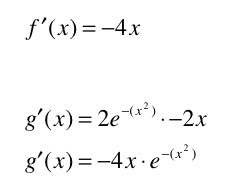## Thursday, June 11, 2009

### A neat application for derivatives

Whoa! May was crazy. But I survived. Here's a fun problem.

The functiongives a Bell curve. The functiongives a downward-opening parabola that fits exactly under the Bell curve, like so:In other words, f(x) is a lower boundary to g(x):How to go about proving this? Algebraically, simplifying the above doesn't do much. It turns out, though, that looking at the derivatives does prove this.Using the Chain Rule for compounded functions on g(x) reveals the similarity between the two functions. Here's a graph of the two derivatives:On the lower right-hand side, quadrant IV, f(x) has a more negative slope than g(x), which is easy enough to prove:On the upper left-hand side, quadrant II, f(x) has a more positive slope than g(x), which has virtually the same proof:(Just remember that the xs in these inequalities are negative, so the inequality signs are flipped twice, back to their original position.)

What does this prove? One final fact: at x = 0, f(0) = g(0).

As x increases, the slope of f(x) is always more negative than the slope of g(x) -- they start equal at x = 0, but f(x) decreases more rapidly, so f(x) must always be less than g(x) as x increases. On the other side, the logic is simply reversed.